• +91 9971497814
• info@interviewmaterial.com

# Polynomials Ex-2.3 Interview Questions Answers

### Related Subjects

Question 1 :

Divide the polynomial p(x) by the polynomialg(x) and find the quotient and remainder in each of the following:

_(_i) p(x) = x3 – 3x2 + 5x – 3, g(x) = x2 – 2

_(ii) p(x) = x4 – 3x2 + 4x + 5, g(x) = x2 + 1 – x
_(iii) p(x) = x4– 5x + 6, g(x) = 2 – x2

(i) p(x) = x3-3x2+5x–3, g(x) = x2–2

Solution:

Here

Dividend = p(x) = x3-3x2+5x–3

Divisor = g(x) = x2– 2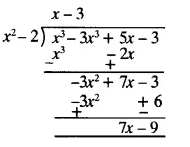Therefore, upon division we get,

Quotient = x–3

Remainder = 7x–9

(ii) p(x) = x4-3x2+4x+5, g(x) = x2+1-x

Solution:

Here

Dividend = p(x) = x– 3x+4x +5

Divisor = g(x) = x2 +1-x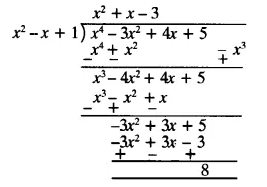Therefore, upon division we get,

Quotient = x+ x–3

Remainder = 8

(iii) p(x) =x4–5x+6, g(x) = 2–x2

Solution:

Here

Dividend = p(x) =x4 – 5x + 6 =x+0x2–5x+6

Divisor = g(x) = 2–x2 = –x2+2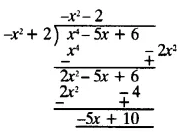Therefore, upon division we get,

Quotient = -x2-2

Remainder = -5x + 10

Question 2 :

Check whether the first polynomial is a factorof the second polynomial by dividing the second polynomial by the firstpolynomial:

_(i) t2 – 3, 2t4 + 3t3 – 2t2– 9t – 12
_(ii) x2 + 3x + 1, 3x4 + 5x3 – 7x2 + 2x + 2

_(iii) x2 + 3x + 1, x5 – 4x+ x2 + 3x + 1

(i) t2-3, 2t+3t3-2t2-9t-12

Solutions:

Here,

First polynomial = t2-3

Second polynomial = 2t+3t3-2t-9t-12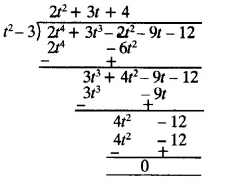As we can see, the remainder is left as 0.Therefore, we say that, t2-3 is a factor of 2t2+3t+4.

(ii)x2+3x+1 , 3x4+5x3-7x2+2x+2

Solutions:

Here,

First polynomial = x2+3x+1

Second polynomial = 3x4+5x3-7x2+2x+2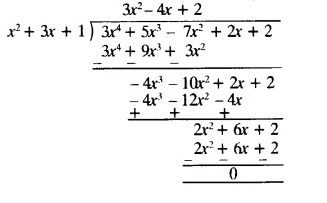As we can see, the remainder is left as 0.Therefore, we say that, x2 + 3x + 1 is a factor of 3x4+5x3-7x2+2x+2.

(iii) x3-3x+1, x5-4x3+x2+3x+1

Solutions:

Here,

First polynomial = x3-3x+1

Second polynomial = x5-4x3+x2+3x+1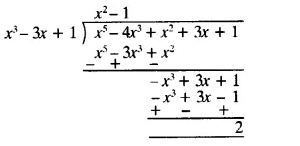As we can see, the remainder is not equal to0. Therefore, we say that, x3-3x+1 is not a factor of x5-4x3+x2+3x+1.

Question 3 :

Obtain all other zeroes of 3x4+6x3-2x2-10x-5,if two of its zeroes are √(5/3) and – √(5/3).

Solutions:

Since this is a polynomial equation of degree4, hence there will be total 4 roots.

√(5/3) and – √(5/3) are zeroes of polynomial f(x).

(x –√(5/3)) (x+√(5/3) =x2-(5/3) = 0

(3x2−5)=0, is a factor of given polynomial f(x).

Now, when we will divide f(x) by (3x2−5)the quotient obtained will also be a factor of f(x) and the remainder will be0.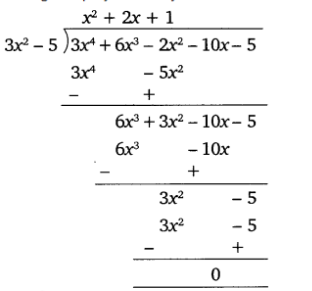Therefore, 3x+6x−2x−10x–5

= (3x–5)(x2+2x+1)

Now, on further factorizing (x2+2x+1)we get,

x2+2x+1=0

x2+x+x+1 = 0

x(x+1)+1(x+1) = 0

(x+1)(x+1) = 0

So, its zeroes are given by: x=−1 and x = −1.

Therefore, all four zeroes of given polynomialequation are:

√(5/3),- √(5/3) , −1 and −1.

Question 4 :

On dividing x3-3x2+x+2 by a polynomial g(x), the quotient and remainder werex–2 and –2x+4, respectively. Find g(x).

Solution:

Here,

Dividend, p(x) = x3-3x2+x+2

Quotient = x-2

Remainder = –2x+4

We have to find the value of Divisor, g(x) =?

As we know,

Dividend = Divisor × Quotient + Remainder

x3-3x2+x+2 = g(x)×(x-2) + (-2x+4)

x3-3x2+x+2-(-2x+4) =g(x)×(x-2)

Therefore, g(x) × (x-2) = x3-3x2+x+2

Now, for finding g(x) we will divide x3-3x2+x+2with (x-2)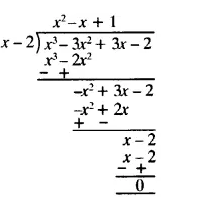Therefore, g(x) = (x2–x+1)

Question 5 :

Give examples of polynomials p(x), g(x), q(x)and r(x), which satisfy the division algorithm and

(i) deg p(x) = deg q(x)

(ii) deg q(x) = deg r(x)

(iii) deg r(x) = 0

Answer 5 :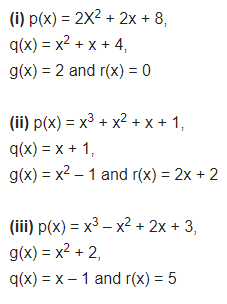Todays Deals### Polynomials Ex-2.3 Contributorskrishan

Name:
Email:

# Latest News# 9000 interview questions in different categories